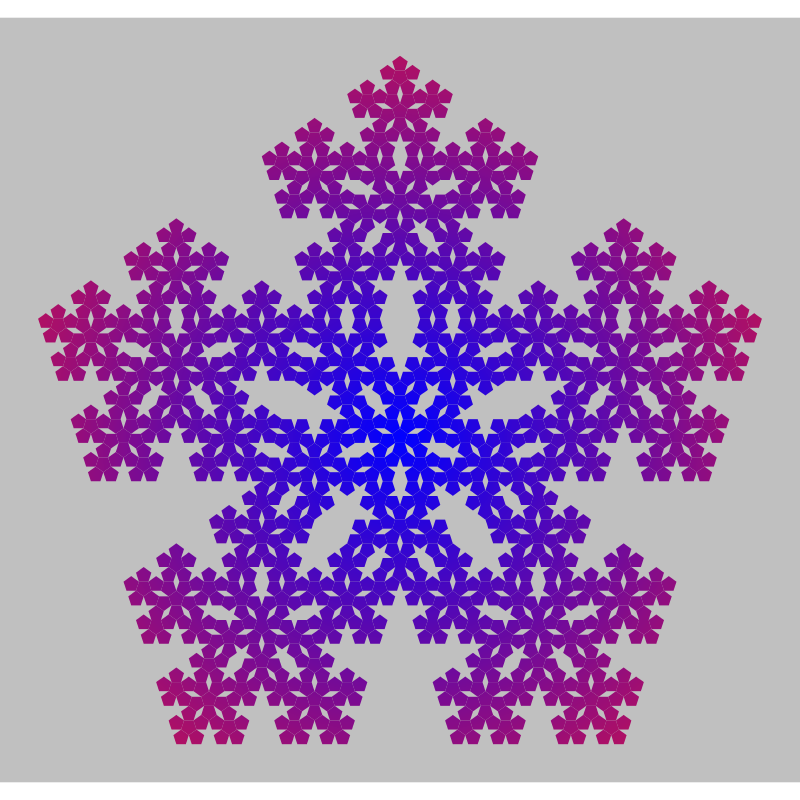Fractal construction with pentagons.Author: Brent Yorgey
> import Diagrams.Backend.SVG.CmdLine
> {-# LANGUAGE NoMonomorphismRestriction #-}
> import Diagrams.Prelude

A $$0$$-pentaflake is just a regular pentagon:

> grad = defaultRG & _RG . rGradStops .~ mkStops [(blue,0,1), (crimson,1,1)]
>                  & _RG . rGradRadius1 .~ 50
> pentaflake' 0 = regPoly 5 1 # lw none

An $$n$$-pentaflake is an $$(n-1)$$-pentaflake surrounded by five more. The appends function is useful here for positioning the five pentaflakes around the central one.

> pentaflake' n = appends
>                   pCenter
>                   (zip vs (repeat (rotateBy (1/2) pOutside)))
>   where vs = iterateN 5 (rotateBy (1/5))
>            . (if odd n then negated else id)
>            \$ unitY
>         pCenter  = pentaflake' (n-1)
>         pOutside = pCenter # opacity (1.7 / fromIntegral n)
>
> pentaflake n = pentaflake' n # fillTexture grad # bgFrame 4 silver

A $$4$$-pentaflake looks nice. Of course there’s an exponential blowup in the number of primitives, so generating higher-order pentaflakes can take a long time!

> example = pentaflake 4
> main = mainWith (example :: Diagram B)